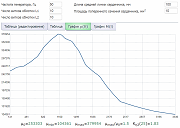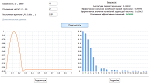Research website of Vyacheslav Gorchilin
Calculate online
• Tesla transformer combined mode calculation. Charge PumpThe combination of two modes of operation of the Tesla transformer coil increases the efficiency of its work, and allows it to work as a pump of charges from the Earth. This calculator is dedicated to the search for optimal parameters for such modes, efficiency and power resulting from such work.
The theoretical background is given in here.
• Calculation of an oscillatory circuit for resonance of the second kindThis calculator calculates the values ​​of the elements and the resonant frequency for the conditions for the existence of a resonance of the second kind. Unlike the classical one, in the resonance of the second kind, not three, but four parameters are taken into account: inductance, capacitance, resistance and frequency.
The theoretical background is given in here.
• Calculation of a parametric generator of the second kind. Parametric inductanceThe purpose of this calculator is to provide a visual representation of transient and stationary processes resulting from parametric generation of electrical energy, and also, - obtaining some initial parameters of a real device: the shape and frequency of oscillations, phase shift between generators, inductance and Q-factor of the coil, load resistance.
The theoretical background is provided by here.
• The graph of dependence of magnetic permeability on intensity of magnetic fieldLaboratory work for obtaining some important parameters and graphs of dependence of magnetic permeability of ferromagnetic materials on the magnetic field. As a result of its holding, the user receives all the necessary data for the construction of real generators and experiments with inductors. In addition to University lab, the calculator computes the theoretically achievable value of increment of efficiency of the second kind for the parametric change of inductance with back EMF.
Theoretical justification of the additional energy from the back EMF in the inductor core is provided here.
• The calculation of the single layer coil on a 1/4 wave taking into account the capacity of the groundThe calculator is designed to calculate the parameters of a single-layer coil by 1/4 wavelength, taking into account the capacity of the ground. Connecting external tanks: the Torah and the condenser, makes it possible and the classical calculation of the Tesla transformer. The lower subsection of this calculator is the precise definition of the capacity of the ground for that coil in your area.
• The combination of the waves in a single layer coilThe new version of the online calculator for combining different types of waves in a single layer inductor. Counts of various coil parameters depending on its design and the material of the frame. It is considered grounding and shielding.
A previous version of this calculator is here.
• Standing waves in long lines. AnimationAnimated representation of the standing waves produced by the excitation of a long line set of waves consisting of several harmonics. Changes the phase and the amplitude of the harmonic, the ratio of the length of the line frequency, q-factor.
• Calculation of pulse pumping Tesla transformerCounts of some output parameters of the Tesla transformer under impulse excitation. The result is displayed relative to the first harmonic from the spectrum obtained in the secondary circuit of the oscillation.
• The calculation of the serial RC or RL circuitCalculator calculates the output parameters: operating voltage, power dissipation and phase of the voltage depending on the input a sinusoidal voltage to RC or RL circuit. Also counted and the value of the reactance of capacitor or inductor for a given frequency.
• The calculation of the dual-frequency series-parallel resonant circuitThe calculator allows you to calculate the parameters of the dual-frequency diagrams of series and parallel resonant circuit online. It is possible to into account the capacity of the ground and has several modes of distribution of waves in the primary coil. There are recommendations for the practical implementation of the device.
• Power supply and capacitor energy balanceThe calculator is designed to calculate the energy balance of the power source and the charged capacitor through the converter circuit, which is a "black box", about the structure of which we know nothing. But we know the power supply data, capacitor data and charging time. Based on this data, we calculate the energy consumed by the power supply for the operation of the converter, and the energy received on the capacitor after the end of charging. By dividing the expended and received energies, we get their balance.
• The free energy back EMF in the inductor coreThe calculator allows to find the optimal parametric dependence of change of inductance of current and mode excitation of the generator to produce maximum efficiency in a closed RL-circuit, where the source of power is back EMF.
Theoretical justification of the additional energy from the back EMF in the inductor core is provided here.
• Circuit simulator onlineThe simulator allows to visualize some of the processes that occur in electronic circuits.
The program is spread under a free license, like education. It is not recommended to use the program to simulate real-world circuits, as many components in it are idealized.
The program supports the following languages: English, Russian, Danish, German and Polish.
• Pass
• The calculation of the current amplifier the parametric inductanceThe calculator is designed to calculate the parameters of different circuit solutions to the current amplifier. His calculations are based on combined parametric circuits of the first and second kind and rather accurately reflect the phenomena occurring there.
Also, the calculator can be used to study transients in parametric and non-parametric RL-circuits.